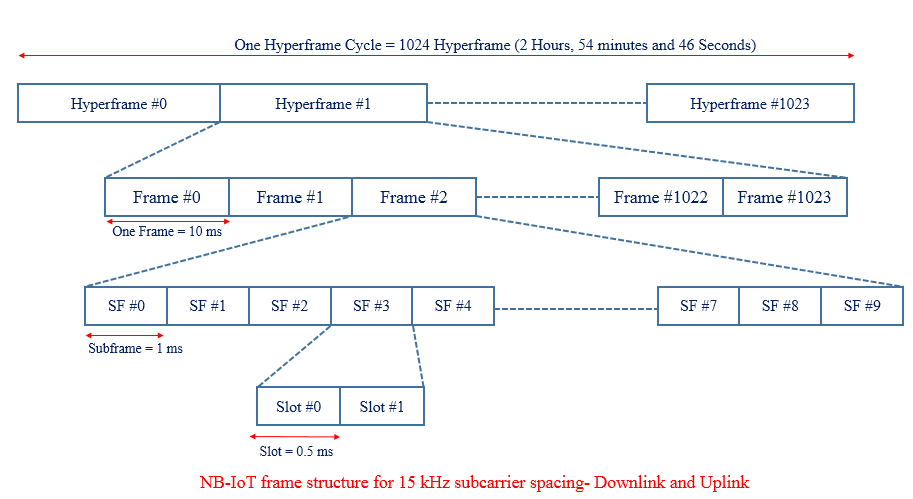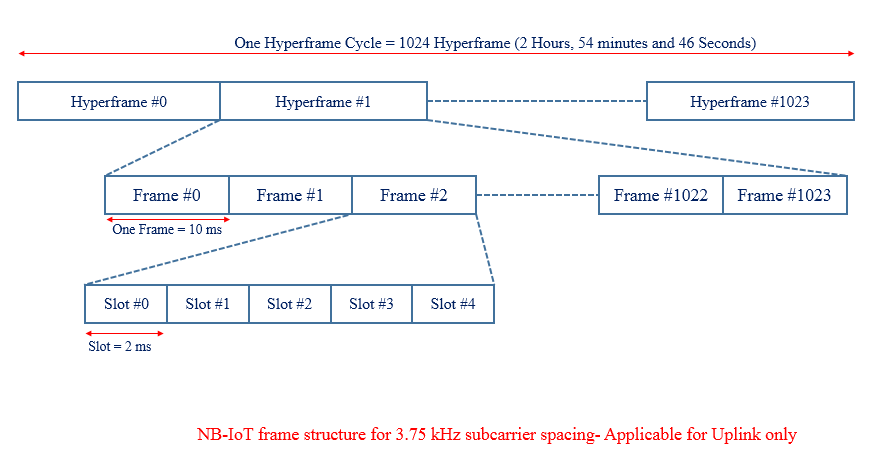# Narrow Band IoT Frame Structure

NB- IoT frame structure is illustrated in the below picture.  At the highest level, it starts with a hyperframe cycle where one hyperframe cycle has 1024 hyper frames and each consisting of 1024 frames.

One frame consists of 10 sub-frames and each subframe is dividable into two slots each of 0.5 ms which is similar to the traditional LTE system. In the DL and UL, the NB-IoT design supports a sub-carrier spacing of 15 KHz, for which each frame contains 20 slots. In the UL, the NB-IoT design supports an additional sub-carrier spacing of 3.75 KHz. For this alternative sub-carrier spacing, each frame is directly divided into five slots, each of 2 ms, there is no subframe concept.Case #2 Uplink Subcarrier Spacing 3.75 KHzNotation and Range:

• Hyperframe:  Hyper System Srame Number (H-SFN), Range  : 0 – 1023
• Frame System Frame Number (SFN), Range  : 0 – 1023
• Subframe: Subframe Number (SN), Range  : 0 – 9

Each subframe can, therefore, be uniquely identified by an H-SFN, an SFN, and a subframe number (SN).

Cycle Duration:

• Duration of a Frame cycle =(No. of Frame in one cycle) x (Duration of one frame)                                                                                                  = 1024 x 10 ms =10240 ms = 10.24 seconds
• Duration of a Hyperframe cycle = (No. of Hyperframe in one cycle) x (Duration of one Hperframe)                                                                                 = 1024 x 10.24 s =104857.60 ms = 2 Hours, 54 minutes and 46 seconds
Recommended
3GPP has defined a set of frequency bands for which…